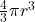# Simple Tetragonal Unit Cell

Alright, now we’re getting to the less-common crystal structures. If you’re searching for information about simple tetragonal crystal cells, I’m assuming you’re a somewhat advanced student in materials science, so I’ll give you the facts quickly and with little explanation. If you are new to materials science and don’t know what something means, you can check out my articles on the FCC, BCC, or HCP crystals, which explain terminology more slowly.

As far as I know, there are no real-world materials that exhibit a simple tetragonal unit cell. If this structure were to exist, it would be a rectangular prism with an atom on each corner. There are “primitive tetragonal” crystals, which exist with multiple atoms that overall display primitive tetragonal symmetry. The simple tetragonal unit cell would belong to space group #123 or P4/mmm, with Pearson symbol tP1. There is no prototype or Strukturbericht for simple tetragonal.

The simple tetragonal unit cell can be imagined as a cube that is slightly taller or shorter in one direction, with an atom on each corner. Pure materials never take this crystal structure, and it exists only mathematically. Simple tetragonal has 1 atom per unit cell, lattice constant a = 2R (a<c) or c = 2R (a>c), Coordination Number CN = 6 (4 or 2), and Atomic Packing Factor APF < 52%.

### Common Examples of Simple Tetragonal Materials

None.

However, there are still materials made of multiple kinds of atoms that have a primitive tetragonal Bravais lattice, such as AuCu.

### Simple Tetragonal Coordination Number

The simple tetragonal unit cell is a distortion of the simple cubic unit cell. You can imagine taking a cube and elongating it, or shrinking it, along one direction.

Simple cubic has 6 nearest-neighbors, so if the distortion is very small, you might consider that all 6 bonds are still the same length, giving CN = 6. However, the true depiction depends on whether the cube is elongated or compressed.

If the tetragon is elongated so that a=b<c, there will be 4 true nearest-neighbors. If the tetragon is shrunk such that a=b>c, there will be 2 true nearest-neighbors.

### Simple Tetragonal Lattice Constants

The tetragon is defined as a parallepiped with two sides (a and b) equal and a third side (c) of different length. All angles are 90º.

The close-packed direction is along the short edge(s) of the tetragon, so either a and b, or c. This close-packed direction would therefore have a length of 2r.

If you wanted to describe the simple tetrahedral crystal with math, you would describe the cell with the vectors:### Simple Tetragonal Atomic Packing Factor

There is no simple answer for the simple tetragonal APF, because the answer changes depending on the a/c ratio.

The volume of a tetragon is.

You know there is ⅛ of an atom on each of the 8 corners of the rhombohedron, which adds to one full atom with a spherical radius of.

Combining these, the### Final Thoughts

The simple tetrahedral crystal structure does not exist in real life, but corresponds to the mathematical concept of the primitive tetrahedral Bravais lattice.

Summary Table

### References and Further Reading

If you want to know more about the basics of crystallography, check out this article about crystals and grains.

If you weren’t sure about the difference between crystal structure and Bravais lattice, check out this article.

I also mentioned atomic packing factor (APF) earlier in this article. This is an important concept in your introductory materials science class, so if you want a full explanation of APF, check out this page.

If you’re interested in advanced crystallography or crystallography databases, you may want to check out the AFLOW crystallographic library.

For a great reference for all crystal structures, check out “The AFLOW Library of Crystallographic Prototypes.”

The 14 Basic Crystal Structures

If you want to learn about specific crystal structures, here is a list of my articles about Bravais lattices and some related crystal structures for pure elements. Simple Tetragonal is one of these 14 Bravais lattices and also occurs as a crystal structure.

Other articles in my crystallography series include: